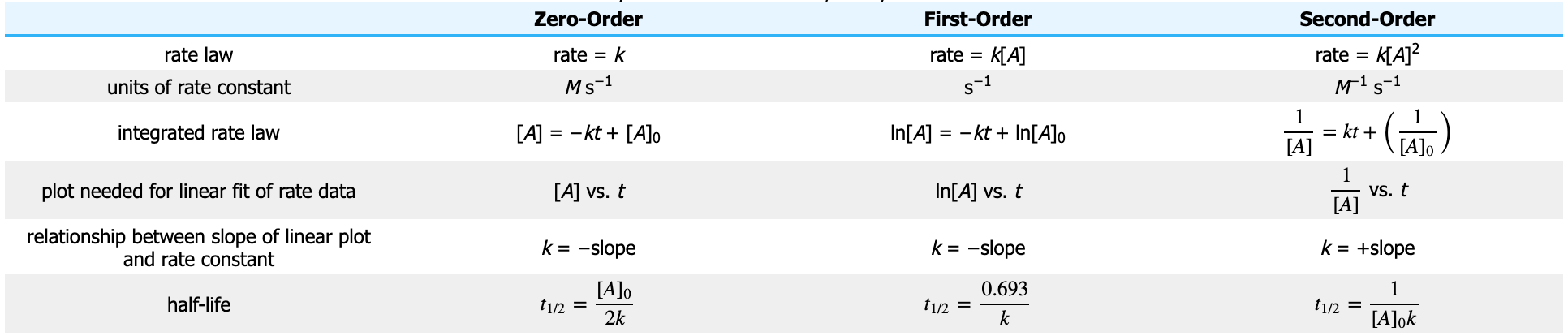# Problem: The following reaction was monitored as a function of time:AB → A+BA plot of 1/[AB] versus time yields a straight line with slope 5.5 x10-2(M•s)-11) What is the value of the rate constant (k) for this reaction at this temperature?2) What is the half-life when the initial concentration is 0.53 M?3) If the initial concentration of AB is 0.270 M, and the reaction mixture initially contains no products, what are the concentrations of A and B after 70 s?

###### FREE Expert Solution

Recall:94% (176 ratings)###### Problem Details

The following reaction was monitored as a function of time:

AB → A+B
A plot of 1/[AB] versus time yields a straight line with slope 5.5 x10-2(M•s)-1

1) What is the value of the rate constant (k) for this reaction at this temperature?

2) What is the half-life when the initial concentration is 0.53 M?

3) If the initial concentration of AB is 0.270 M, and the reaction mixture initially contains no products, what are the concentrations of A and B after 70 s?